Publicité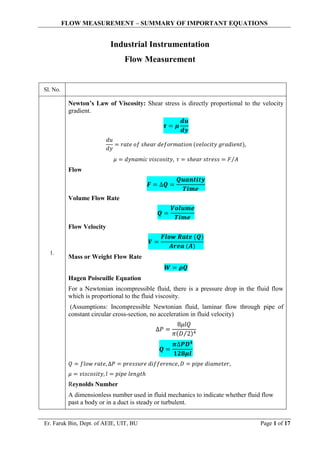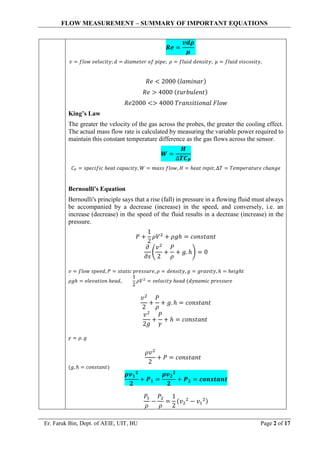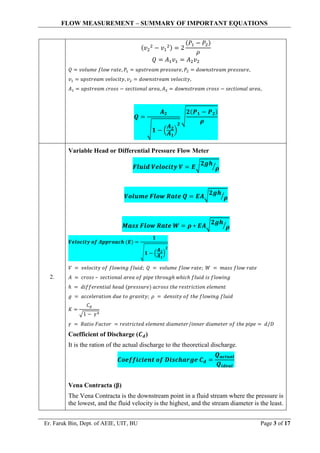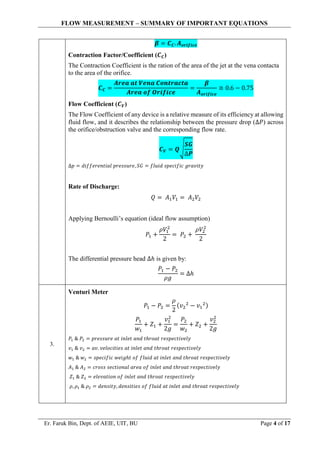Publicité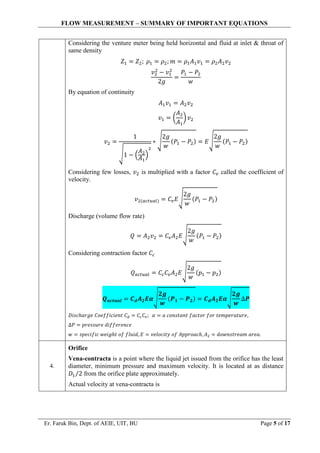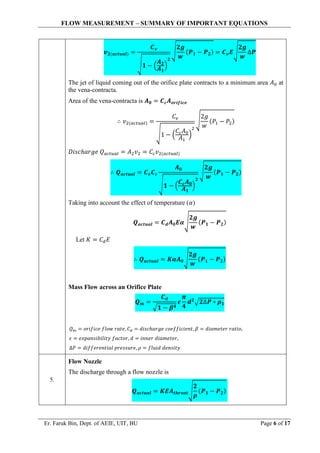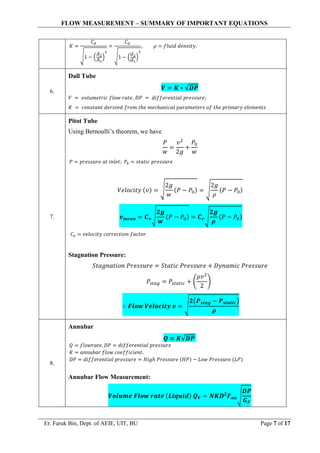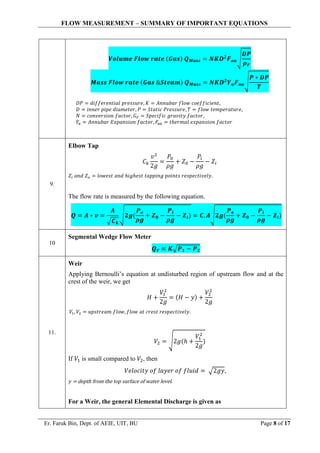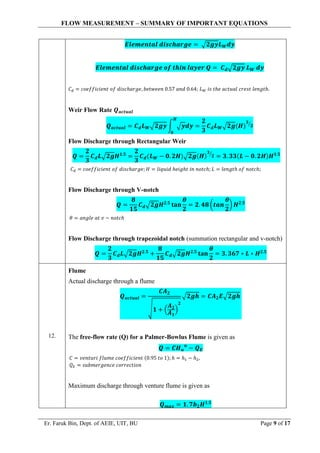Publicité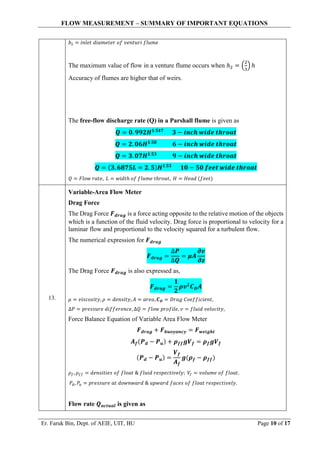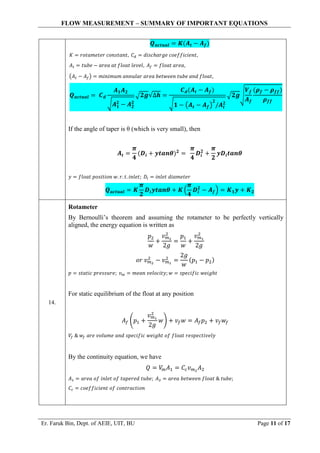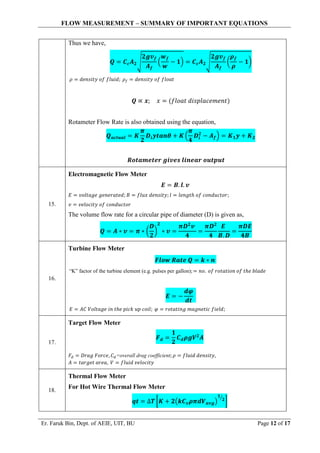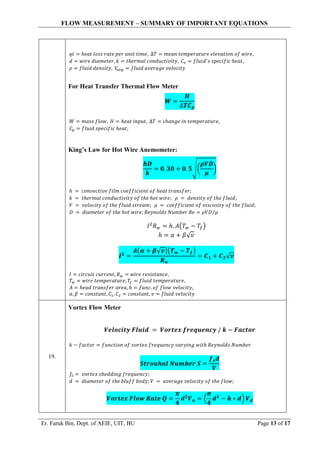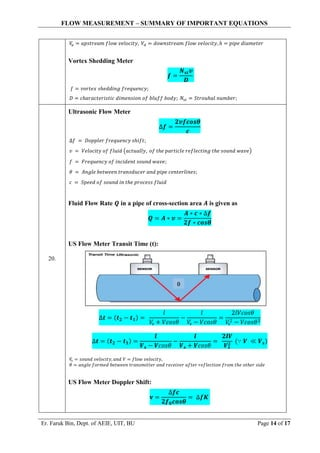Publicité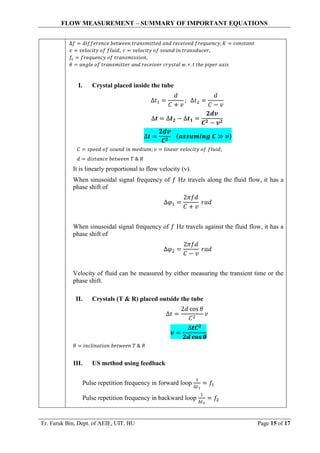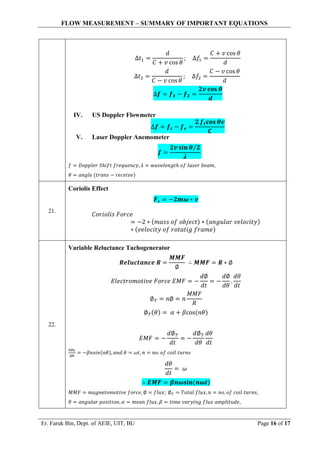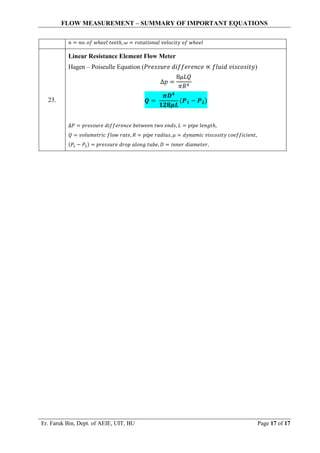Prochain SlideShare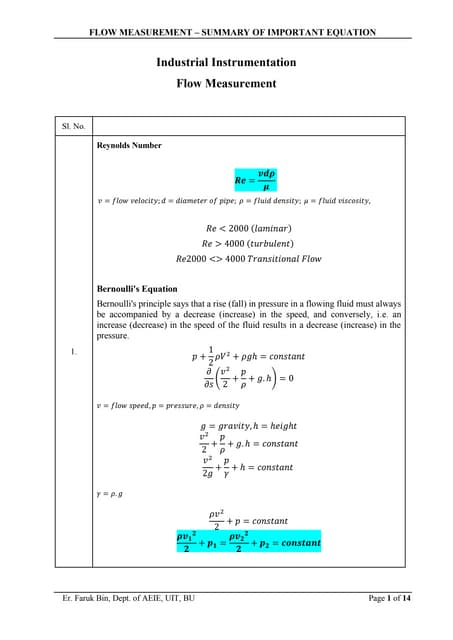Industrial instrumentation flow measurement important equations
Chargement dans ... 3
1 sur 17
Publicité

### Eqautions_1_Industrial Instrumentation - Flow Measurement Important Equations.pdf

1. FLOW MEASUREMENT – SUMMARY OF IMPORTANT EQUATIONS Er. Faruk Bin, Dept. of AEIE, UIT, BU Page 1 of 17 Industrial Instrumentation Flow Measurement Sl. No. 1. Newton’s Law of Viscosity: Shear stress is directly proportional to the velocity gradient. 𝝉 = 𝝁 𝒅𝒖 𝒅𝒚 𝑑𝑢 𝑑𝑦 = 𝑟𝑎𝑡𝑒 𝑜𝑓 𝑠ℎ𝑒𝑎𝑟 𝑑𝑒𝑓𝑜𝑟𝑚𝑎𝑡𝑖𝑜𝑛 (𝑣𝑒𝑙𝑜𝑐𝑖𝑡𝑦 𝑔𝑟𝑎𝑑𝑖𝑒𝑛𝑡), 𝜇 = 𝑑𝑦𝑛𝑎𝑚𝑖𝑐 𝑣𝑖𝑠𝑐𝑜𝑠𝑖𝑡𝑦, 𝜏 = 𝑠ℎ𝑒𝑎𝑟 𝑠𝑡𝑟𝑒𝑠𝑠 = 𝐹 𝐴 ⁄ Flow 𝑭 = ∆𝑸 = 𝑸𝒖𝒂𝒏𝒕𝒊𝒕𝒚 𝑻𝒊𝒎𝒆 Volume Flow Rate 𝑸 = 𝑽𝒐𝒍𝒖𝒎𝒆 𝑻𝒊𝒎𝒆 Flow Velocity 𝑽 = 𝑭𝒍𝒐𝒘 𝑹𝒂𝒕𝒆 (𝑸) 𝑨𝒓𝒆𝒂 (𝑨) Mass or Weight Flow Rate 𝑾 = 𝝆𝑸 Hagen Poiseuille Equation For a Newtonian incompressible fluid, there is a pressure drop in the fluid flow which is proportional to the fluid viscosity. (Assumptions: Incompressible Newtonian fluid, laminar flow through pipe of constant circular cross-section, no acceleration in fluid velocity) ∆𝑃 = 8𝜇𝑙𝑄 𝜋(𝐷 2 ⁄ )4 𝑸 = 𝝅∆𝑷𝑫𝟒 𝟏𝟐𝟖𝝁𝒍 𝑄 = 𝑓𝑙𝑜𝑤 𝑟𝑎𝑡𝑒, ∆𝑃 = 𝑝𝑟𝑒𝑠𝑠𝑢𝑟𝑒 𝑑𝑖𝑓𝑓𝑒𝑟𝑒𝑛𝑐𝑒, 𝐷 = 𝑝𝑖𝑝𝑒 𝑑𝑖𝑎𝑚𝑒𝑡𝑒𝑟, 𝜇 = 𝑣𝑖𝑠𝑐𝑜𝑠𝑖𝑡𝑦, 𝑙 = 𝑝𝑖𝑝𝑒 𝑙𝑒𝑛𝑔𝑡ℎ Reynolds Number A dimensionless number used in fluid mechanics to indicate whether fluid flow past a body or in a duct is steady or turbulent.
2. FLOW MEASUREMENT – SUMMARY OF IMPORTANT EQUATIONS Er. Faruk Bin, Dept. of AEIE, UIT, BU Page 2 of 17 𝑹𝒆 = 𝒗𝒅𝝆 𝝁 𝑣 = 𝑓𝑙𝑜𝑤 𝑣𝑒𝑙𝑜𝑐𝑖𝑡𝑦; 𝑑 = 𝑑𝑖𝑎𝑚𝑒𝑡𝑒𝑟 𝑜𝑓 𝑝𝑖𝑝𝑒; 𝜌 = 𝑓𝑙𝑢𝑖𝑑 𝑑𝑒𝑛𝑠𝑖𝑡𝑦; 𝜇 = 𝑓𝑙𝑢𝑖𝑑 𝑣𝑖𝑠𝑐𝑜𝑠𝑖𝑡𝑦, 𝑅𝑒 < 2000 (𝑙𝑎𝑚𝑖𝑛𝑎𝑟) 𝑅𝑒 > 4000 (𝑡𝑢𝑟𝑏𝑢𝑙𝑒𝑛𝑡) 𝑅𝑒2000 <> 4000 𝑇𝑟𝑎𝑛𝑠𝑖𝑡𝑖𝑜𝑛𝑎𝑙 𝐹𝑙𝑜𝑤 King’s Law The greater the velocity of the gas across the probes, the greater the cooling effect. The actual mass flow rate is calculated by measuring the variable power required to maintain this constant temperature difference as the gas flows across the sensor. 𝑾 = 𝑯 ∆𝑻𝑪𝑷 𝐶𝑃 = 𝑠𝑝𝑒𝑐𝑖𝑓𝑖𝑐 ℎ𝑒𝑎𝑡 𝑐𝑎𝑝𝑎𝑐𝑖𝑡𝑦, 𝑊 = 𝑚𝑎𝑠𝑠 𝑓𝑙𝑜𝑤, 𝐻 = ℎ𝑒𝑎𝑡 𝑖𝑛𝑝𝑖𝑡, ∆𝑇 = 𝑇𝑒𝑚𝑝𝑒𝑟𝑎𝑡𝑢𝑟𝑒 𝑐ℎ𝑎𝑛𝑔𝑒 Bernoulli's Equation Bernoulli's principle says that a rise (fall) in pressure in a flowing fluid must always be accompanied by a decrease (increase) in the speed, and conversely, i.e. an increase (decrease) in the speed of the fluid results in a decrease (increase) in the pressure. 𝑃 + 1 2 𝜌𝑉2 + 𝜌𝑔ℎ = 𝑐𝑜𝑛𝑠𝑡𝑎𝑛𝑡 𝜕 𝜕𝑠 ( 𝑣2 2 + 𝑃 𝜌 + 𝑔. ℎ) = 0 𝑣 = 𝑓𝑙𝑜𝑤 𝑠𝑝𝑒𝑒𝑑, 𝑃 = 𝑠𝑡𝑎𝑡𝑖𝑐 𝑝𝑟𝑒𝑠𝑠𝑢𝑟𝑒, 𝜌 = 𝑑𝑒𝑛𝑠𝑖𝑡𝑦, 𝑔 = 𝑔𝑟𝑎𝑣𝑖𝑡𝑦, ℎ = ℎ𝑒𝑖𝑔ℎ𝑡 𝜌𝑔ℎ = 𝑒𝑙𝑒𝑣𝑎𝑡𝑖𝑜𝑛 ℎ𝑒𝑎𝑑, 1 2 𝜌𝑉2 = 𝑣𝑒𝑙𝑜𝑐𝑖𝑡𝑦 ℎ𝑒𝑎𝑑 (𝑑𝑦𝑛𝑎𝑚𝑖𝑐 𝑝𝑟𝑒𝑠𝑠𝑢𝑟𝑒 𝑣2 2 + 𝑃 𝜌 + 𝑔. ℎ = 𝑐𝑜𝑛𝑠𝑡𝑎𝑛𝑡 𝑣2 2𝑔 + 𝑃 𝛾 + ℎ = 𝑐𝑜𝑛𝑠𝑡𝑎𝑛𝑡 𝛾 = 𝜌. 𝑔 𝜌𝑣2 2 + 𝑃 = 𝑐𝑜𝑛𝑠𝑡𝑎𝑛𝑡 (𝑔, ℎ = 𝑐𝑜𝑛𝑠𝑡𝑎𝑛𝑡) 𝝆𝒗𝟏 𝟐 𝟐 + 𝑷𝟏 = 𝝆𝒗𝟐 𝟐 𝟐 + 𝑷𝟐 = 𝒄𝒐𝒏𝒔𝒕𝒂𝒏𝒕 𝑃1 𝜌 − 𝑃2 𝜌 = 1 2 (𝑣2 2 − 𝑣1 2)
3. FLOW MEASUREMENT – SUMMARY OF IMPORTANT EQUATIONS Er. Faruk Bin, Dept. of AEIE, UIT, BU Page 3 of 17 (𝑣2 2 − 𝑣1 2) = 2 (𝑃1 − 𝑃2) 𝜌 𝑄 = 𝐴1𝑣1 = 𝐴2𝑣2 𝑄 = 𝑣𝑜𝑙𝑢𝑚𝑒 𝑓𝑙𝑜𝑤 𝑟𝑎𝑡𝑒, 𝑃1 = 𝑢𝑝𝑠𝑡𝑟𝑒𝑎𝑚 𝑝𝑟𝑒𝑠𝑠𝑢𝑟𝑒, 𝑃2 = 𝑑𝑜𝑤𝑛𝑠𝑡𝑟𝑒𝑎𝑚 𝑝𝑟𝑒𝑠𝑠𝑢𝑟𝑒, 𝑣1 = 𝑢𝑝𝑠𝑡𝑟𝑒𝑎𝑚 𝑣𝑒𝑙𝑜𝑐𝑖𝑡𝑦, 𝑣2 = 𝑑𝑜𝑤𝑛𝑠𝑡𝑟𝑒𝑎𝑚 𝑣𝑒𝑙𝑜𝑐𝑖𝑡𝑦, 𝐴1 = 𝑢𝑝𝑠𝑡𝑟𝑒𝑎𝑚 𝑐𝑟𝑜𝑠𝑠 − 𝑠𝑒𝑐𝑡𝑖𝑜𝑛𝑎𝑙 𝑎𝑟𝑒𝑎, 𝐴2 = 𝑑𝑜𝑤𝑛𝑠𝑡𝑟𝑒𝑎𝑚 𝑐𝑟𝑜𝑠𝑠 − 𝑠𝑒𝑐𝑡𝑖𝑜𝑛𝑎𝑙 𝑎𝑟𝑒𝑎, 𝑸 = 𝑨𝟐 √𝟏 − ( 𝑨𝟐 𝑨𝟏 ) 𝟐 √ 𝟐(𝑷𝟏 − 𝑷𝟐) 𝝆 2. Variable Head or Differential Pressure Flow Meter 𝑭𝒍𝒖𝒊𝒅 𝑽𝒆𝒍𝒐𝒄𝒊𝒕𝒚 𝑽 = 𝑬√𝟐𝒈𝒉 𝝆 ⁄ 𝑽𝒐𝒍𝒖𝒎𝒆 𝑭𝒍𝒐𝒘 𝑹𝒂𝒕𝒆 𝑸 = 𝑬𝑨√𝟐𝒈𝒉 𝝆 ⁄ 𝑴𝒂𝒔𝒔 𝑭𝒍𝒐𝒘 𝑹𝒂𝒕𝒆 𝑾 = 𝝆 ∗ 𝑬𝑨√𝟐𝒈𝒉 𝝆 ⁄ 𝑽𝒆𝒍𝒐𝒄𝒊𝒕𝒚 𝒐𝒇 𝑨𝒑𝒑𝒓𝒐𝒂𝒄𝒉 (𝑬) = 𝟏 √𝟏 − ( 𝑨𝟐 𝑨𝟏 ) 𝟐 𝑉 = 𝑣𝑒𝑙𝑜𝑐𝑖𝑡𝑦 𝑜𝑓 𝑓𝑙𝑜𝑤𝑖𝑛𝑔 𝑓𝑙𝑢𝑖𝑑; 𝑄 = 𝑣𝑜𝑙𝑢𝑚𝑒 𝑓𝑙𝑜𝑤 𝑟𝑎𝑡𝑒; 𝑊 = 𝑚𝑎𝑠𝑠 𝑓𝑙𝑜𝑤 𝑟𝑎𝑡𝑒 𝐴 = 𝑐𝑟𝑜𝑠𝑠 – 𝑠𝑒𝑐𝑡𝑖𝑜𝑛𝑎𝑙 𝑎𝑟𝑒𝑎 𝑜𝑓 𝑝𝑖𝑝𝑒 𝑡ℎ𝑟𝑜𝑢𝑔ℎ 𝑤ℎ𝑖𝑐ℎ 𝑓𝑙𝑢𝑖𝑑 𝑖𝑠 𝑓𝑙𝑜𝑤𝑖𝑛𝑔 ℎ = 𝑑𝑖𝑓𝑓𝑒𝑟𝑒𝑛𝑡𝑖𝑎𝑙 ℎ𝑒𝑎𝑑 (𝑝𝑟𝑒𝑠𝑠𝑢𝑟𝑒) 𝑎𝑐𝑟𝑜𝑠𝑠 𝑡ℎ𝑒 𝑟𝑒𝑠𝑡𝑟𝑖𝑐𝑡𝑖𝑜𝑛 𝑒𝑙𝑒𝑚𝑒𝑛𝑡 𝑔 = 𝑎𝑐𝑐𝑒𝑙𝑒𝑟𝑎𝑡𝑖𝑜𝑛 𝑑𝑢𝑒 𝑡𝑜 𝑔𝑟𝑎𝑣𝑖𝑡𝑦; 𝜌 = 𝑑𝑒𝑛𝑠𝑖𝑡𝑦 𝑜𝑓 𝑡ℎ𝑒 𝑓𝑙𝑜𝑤𝑖𝑛𝑔 𝑓𝑙𝑢𝑖𝑑 𝐾 = 𝐶𝑑 √1 − 𝛾4 𝛾 = 𝑅𝑎𝑡𝑖𝑜 𝐹𝑎𝑐𝑡𝑜𝑟 = 𝑟𝑒𝑠𝑡𝑟𝑖𝑐𝑡𝑒𝑑 𝑒𝑙𝑒𝑚𝑒𝑛𝑡 𝑑𝑖𝑎𝑚𝑒𝑡𝑒𝑟/𝑖𝑛𝑛𝑒𝑟 𝑑𝑖𝑎𝑚𝑒𝑡𝑒𝑟 𝑜𝑓 𝑡ℎ𝑒 𝑝𝑖𝑝𝑒 = 𝑑/𝐷 Coefficient of Discharge (𝑪𝒅) It is the ration of the actual discharge to the theoretical discharge. 𝑪𝒐𝒆𝒇𝒇𝒊𝒄𝒊𝒆𝒏𝒕 𝒐𝒇 𝑫𝒊𝒔𝒄𝒉𝒂𝒓𝒈𝒆 𝑪𝒅 = 𝑸𝒂𝒄𝒕𝒖𝒂𝒍 𝑸𝒊𝒅𝒆𝒂𝒍 Vena Contracta (β) The Vena Contracta is the downstream point in a fluid stream where the pressure is the lowest, and the fluid velocity is the highest, and the stream diameter is the least.
4. FLOW MEASUREMENT – SUMMARY OF IMPORTANT EQUATIONS Er. Faruk Bin, Dept. of AEIE, UIT, BU Page 4 of 17 𝜷 = 𝑪𝑪. 𝑨𝒐𝒓𝒊𝒇𝒊𝒄𝒆 Contraction Factor/Coefficient (𝑪𝑪) The Contraction Coefficient is the ration of the area of the jet at the vena contacta to the area of the orifice. 𝑪𝑪 = 𝑨𝒓𝒆𝒂 𝒂𝒕 𝑽𝒆𝒏𝒂 𝑪𝒐𝒏𝒕𝒓𝒂𝒄𝒕𝒂 𝑨𝒓𝒆𝒂 𝒐𝒇 𝑶𝒓𝒊𝒇𝒊𝒄𝒆 = 𝜷 𝑨𝒐𝒓𝒊𝒇𝒊𝒄𝒆 ≅ 0.6 − 0.75 Flow Coefficient (𝑪𝑽) The Flow Coefficient of any device is a relative measure of its efficiency at allowing fluid flow, and it describes the relationship between the pressure drop (∆𝑃) across the orifice/obstruction valve and the corresponding flow rate. 𝑪𝑽 = 𝑸√ 𝑺𝑮 ∆𝑷 ∆𝑝 = 𝑑𝑖𝑓𝑓𝑒𝑟𝑒𝑛𝑡𝑖𝑎𝑙 𝑝𝑟𝑒𝑠𝑠𝑢𝑟𝑒, 𝑆𝐺 = 𝑓𝑙𝑢𝑖𝑑 𝑠𝑝𝑒𝑐𝑖𝑓𝑖𝑐 𝑔𝑟𝑎𝑣𝑖𝑡𝑦 Rate of Discharge: 𝑄 = 𝐴1𝑉1 = 𝐴2𝑉2 Applying Bernoulli’s equation (ideal flow assumption) 𝑃1 + 𝜌𝑉1 2 2 = 𝑃2 + 𝜌𝑉2 2 2 The differential pressure head ∆ℎ is given by: 𝑃1 − 𝑃2 𝜌𝑔 = ∆ℎ 3. Venturi Meter 𝑃1 − 𝑃2 = 𝜌 2 (𝑣2 2 − 𝑣1 2) 𝑃1 𝑤1 + 𝑍1 + 𝑣1 2 2𝑔 = 𝑃2 𝑤2 + 𝑍2 + 𝑣2 2 2𝑔 𝑃1 & 𝑃2 = 𝑝𝑟𝑒𝑠𝑠𝑢𝑟𝑒 𝑎𝑡 𝑖𝑛𝑙𝑒𝑡 𝑎𝑛𝑑 𝑡ℎ𝑟𝑜𝑎𝑡 𝑟𝑒𝑠𝑝𝑒𝑐𝑡𝑖𝑣𝑒𝑙𝑦 𝑣1 & 𝑣2 = 𝑎𝑣. 𝑣𝑒𝑙𝑜𝑐𝑖𝑡𝑖𝑒𝑠 𝑎𝑡 𝑖𝑛𝑙𝑒𝑡 𝑎𝑛𝑑 𝑡ℎ𝑟𝑜𝑎𝑡 𝑟𝑒𝑠𝑝𝑒𝑐𝑡𝑖𝑣𝑒𝑙𝑦 𝑤1 & 𝑤2 = 𝑠𝑝𝑒𝑐𝑖𝑓𝑖𝑐 𝑤𝑒𝑖𝑔ℎ𝑡 𝑜𝑓 𝑓𝑙𝑢𝑖𝑑 𝑎𝑡 𝑖𝑛𝑙𝑒𝑡 𝑎𝑛𝑑 𝑡ℎ𝑟𝑜𝑎𝑡 𝑟𝑒𝑠𝑝𝑒𝑐𝑡𝑖𝑣𝑒𝑙𝑦 𝐴1 & 𝐴2 = 𝑐𝑟𝑜𝑠𝑠 𝑠𝑒𝑐𝑡𝑖𝑜𝑛𝑎𝑙 𝑎𝑟𝑒𝑎 𝑜𝑓 𝑖𝑛𝑙𝑒𝑡 𝑎𝑛𝑑 𝑡ℎ𝑟𝑜𝑎𝑡 𝑟𝑒𝑠𝑝𝑒𝑐𝑡𝑖𝑣𝑒𝑙𝑦 𝑍1 & 𝑍2 = 𝑒𝑙𝑒𝑣𝑎𝑡𝑖𝑜𝑛 𝑜𝑓 𝑖𝑛𝑙𝑒𝑡 𝑎𝑛𝑑 𝑡ℎ𝑟𝑜𝑎𝑡 𝑟𝑒𝑠𝑝𝑒𝑐𝑡𝑖𝑣𝑒𝑙𝑦 𝜌, 𝜌1 & 𝜌2 = 𝑑𝑒𝑛𝑠𝑖𝑡𝑦, 𝑑𝑒𝑛𝑠𝑖𝑡𝑖𝑒𝑠 𝑜𝑓 𝑓𝑙𝑢𝑖𝑑 𝑎𝑡 𝑖𝑛𝑙𝑒𝑡 𝑎𝑛𝑑 𝑡ℎ𝑟𝑜𝑎𝑡 𝑟𝑒𝑠𝑝𝑒𝑐𝑡𝑖𝑣𝑒𝑙𝑦
5. FLOW MEASUREMENT – SUMMARY OF IMPORTANT EQUATIONS Er. Faruk Bin, Dept. of AEIE, UIT, BU Page 5 of 17 Considering the venture meter being held horizontal and fluid at inlet & throat of same density 𝑍1 = 𝑍2; 𝜌1 = 𝜌2; 𝑚 = 𝜌1𝐴1𝑣1 = 𝜌2𝐴2𝑣2 𝑣2 2 − 𝑣1 2 2𝑔 = 𝑃1 − 𝑃2 𝑤 By equation of continuity 𝐴1𝑣1 = 𝐴2𝑣2 𝑣1 = ( 𝐴2 𝐴1 ) 𝑣2 𝑣2 = 1 √1 − ( 𝐴2 𝐴1 ) 2 ∗ √ 2𝑔 𝑤 (𝑃1 − 𝑃2) = 𝐸√ 2𝑔 𝑤 (𝑃1 − 𝑃2) Considering few losses, 𝑣2 is multiplied with a factor 𝐶𝑣 called the coefficient of velocity. 𝑣2(𝑎𝑐𝑡𝑢𝑎𝑙) = 𝐶𝑣𝐸√ 2𝑔 𝑤 (𝑃1 − 𝑃2) Discharge (volume flow rate) 𝑄 = 𝐴2𝑣2 = 𝐶𝑣𝐴2𝐸√ 2𝑔 𝑤 (𝑃1 − 𝑃2) Considering contraction factor 𝐶𝑐 𝑄𝑎𝑐𝑡𝑢𝑎𝑙 = 𝐶𝑐𝐶𝑣𝐴2𝐸√ 2𝑔 𝑤 (𝑝1 − 𝑝2) 𝑸𝒂𝒄𝒕𝒖𝒂𝒍 = 𝑪𝒅𝑨𝟐𝑬𝜶√ 𝟐𝒈 𝒘 (𝑷𝟏 − 𝑷𝟐) = 𝑪𝒅𝑨𝟐𝑬𝜶√ 𝟐𝒈 𝒘 ∆𝑷 𝐷𝑖𝑠𝑐ℎ𝑎𝑟𝑔𝑒 𝐶𝑜𝑒𝑓𝑓𝑖𝑐𝑖𝑒𝑛𝑡 𝐶𝑑 = 𝐶𝑐𝐶𝑣; 𝛼 = 𝑎 𝑐𝑜𝑛𝑠𝑡𝑎𝑛𝑡 𝑓𝑎𝑐𝑡𝑜𝑟 𝑓𝑜𝑟 𝑡𝑒𝑚𝑝𝑒𝑟𝑎𝑡𝑢𝑟𝑒, ∆𝑃 = 𝑝𝑟𝑒𝑠𝑠𝑢𝑟𝑒 𝑑𝑖𝑓𝑓𝑒𝑟𝑒𝑛𝑐𝑒 𝑤 = 𝑠𝑝𝑒𝑐𝑖𝑓𝑖𝑐 𝑤𝑒𝑖𝑔ℎ𝑡 𝑜𝑓 𝑓𝑙𝑢𝑖𝑑, 𝐸 = 𝑣𝑒𝑙𝑜𝑐𝑖𝑡𝑦 𝑜𝑓 𝐴𝑝𝑝𝑟𝑜𝑎𝑐ℎ, 𝐴2 = 𝑑𝑜𝑤𝑛𝑠𝑡𝑟𝑒𝑎𝑚 𝑎𝑟𝑒𝑎. 4. Orifice Vena-contracta is a point where the liquid jet issued from the orifice has the least diameter, minimum pressure and maximum velocity. It is located at as distance 𝐷1 2 ⁄ from the orifice plate approximately. Actual velocity at vena-contracta is
6. FLOW MEASUREMENT – SUMMARY OF IMPORTANT EQUATIONS Er. Faruk Bin, Dept. of AEIE, UIT, BU Page 6 of 17 𝒗𝟐(𝒂𝒄𝒕𝒖𝒂𝒍) = 𝑪𝒗 √𝟏 − ( 𝑨𝟐 𝑨𝟏 ) 𝟐 √ 𝟐𝒈 𝒘 (𝑷𝟏 − 𝑷𝟐) = 𝑪𝒗𝑬√ 𝟐𝒈 𝒘 ∆𝑷 The jet of liquid coming out of the orifice plate contracts to a minimum area 𝐴0 at the vena-contracta. Area of the vena-contracta is 𝑨𝟎 = 𝑪𝒄𝑨𝒐𝒓𝒊𝒇𝒊𝒄𝒆 ∴ 𝑣2(𝑎𝑐𝑡𝑢𝑎𝑙) = 𝐶𝑣 √1 − ( 𝐶𝑐𝐴0 𝐴1 ) 2 √ 2𝑔 𝑤 (𝑃1 − 𝑃2) 𝐷𝑖𝑠𝑐ℎ𝑎𝑟𝑔𝑒 𝑄𝑎𝑐𝑡𝑢𝑎𝑙 = 𝐴2𝑣2 = 𝐶𝑐𝑣2(𝑎𝑐𝑡𝑢𝑎𝑙) ∴ 𝑸𝒂𝒄𝒕𝒖𝒂𝒍 = 𝑪𝒗𝑪𝒄 𝑨𝟎 √𝟏 − ( 𝑪𝒄𝑨𝟎 𝑨𝟏 ) 𝟐 √ 𝟐𝒈 𝒘 (𝑷𝟏 − 𝑷𝟐) Taking into account the effect of temperature (𝛼) 𝑸𝒂𝒄𝒕𝒖𝒂𝒍 = 𝑪𝒅𝑨𝟎𝑬𝜶√ 𝟐𝒈 𝒘 (𝑷𝟏 − 𝑷𝟐) Let 𝐾 = 𝐶𝑑𝐸 ∴ 𝑸𝒂𝒄𝒕𝒖𝒂𝒍 = 𝑲𝜶𝑨𝟎√ 𝟐𝒈 𝒘 (𝑷𝟏 − 𝑷𝟐) Mass Flow across an Orifice Plate 𝑸𝒎 = 𝑪𝒅 √𝟏 − 𝜷𝟒 𝝐 𝝅 𝟒 𝒅𝟐 √𝟐∆𝑷 ∗ 𝝆𝟏 𝑄𝑚 = 𝑜𝑟𝑖𝑓𝑖𝑐𝑒 𝑓𝑙𝑜𝑤 𝑟𝑎𝑡𝑒, 𝐶𝑑 = 𝑑𝑖𝑠𝑐ℎ𝑎𝑟𝑔𝑒 𝑐𝑜𝑒𝑓𝑓𝑖𝑐𝑖𝑒𝑛𝑡, 𝛽 = 𝑑𝑖𝑎𝑚𝑒𝑡𝑒𝑟 𝑟𝑎𝑡𝑖𝑜, 𝜖 = 𝑒𝑥𝑝𝑎𝑛𝑠𝑖𝑏𝑖𝑙𝑖𝑡𝑦 𝑓𝑎𝑐𝑡𝑜𝑟, 𝑑 = 𝑖𝑛𝑛𝑒𝑟 𝑑𝑖𝑎𝑚𝑒𝑡𝑒𝑟, ∆𝑃 = 𝑑𝑖𝑓𝑓𝑒𝑟𝑒𝑛𝑡𝑖𝑎𝑙 𝑝𝑟𝑒𝑠𝑠𝑢𝑟𝑒, 𝜌 = 𝑓𝑙𝑢𝑖𝑑 𝑑𝑒𝑛𝑠𝑖𝑡𝑦 5. Flow Nozzle The discharge through a flow nozzle is 𝑸𝒂𝒄𝒕𝒖𝒂𝒍 = 𝑲𝑬𝑨𝒕𝒉𝒓𝒐𝒂𝒕√ 𝟐 𝝆 (𝑷𝟏 − 𝑷𝟐)
7. FLOW MEASUREMENT – SUMMARY OF IMPORTANT EQUATIONS Er. Faruk Bin, Dept. of AEIE, UIT, BU Page 7 of 17 𝐾 = 𝐶𝑑 √1 − ( 𝐴2 𝐴1 ) 2 = 𝐶𝑑 √1 − ( 𝑑2 𝑑1 ) 2 , 𝜌 = 𝑓𝑙𝑢𝑖𝑑 𝑑𝑒𝑛𝑠𝑖𝑡𝑦. 6. Dall Tube 𝑽 = 𝑲 ∗ √𝑫𝑷 𝑉 = 𝑣𝑜𝑙𝑢𝑚𝑒𝑡𝑟𝑖𝑐 𝑓𝑙𝑜𝑤 𝑟𝑎𝑡𝑒, 𝐷𝑃 = 𝑑𝑖𝑓𝑓𝑒𝑟𝑒𝑛𝑡𝑖𝑎𝑙 𝑝𝑟𝑒𝑠𝑠𝑢𝑟𝑒; 𝐾 = 𝑐𝑜𝑛𝑠𝑡𝑎𝑛𝑡 𝑑𝑒𝑟𝑖𝑣𝑒𝑑 𝑓𝑟𝑜𝑚 𝑡ℎ𝑒 𝑚𝑒𝑐ℎ𝑎𝑛𝑖𝑐𝑎𝑙 𝑝𝑎𝑟𝑎𝑚𝑒𝑡𝑒𝑟𝑠 𝑜𝑓 𝑡ℎ𝑒 𝑝𝑟𝑖𝑚𝑎𝑟𝑦 𝑒𝑙𝑒𝑚𝑒𝑛𝑡𝑠 7. Pitot Tube Using Bernoulli’s theorem, we have 𝑃 𝑤 = 𝑣2 2𝑔 + 𝑃0 𝑤 𝑃 = 𝑝𝑟𝑒𝑠𝑠𝑢𝑟𝑒 𝑎𝑡 𝑖𝑛𝑙𝑒𝑡; 𝑃0 = 𝑠𝑡𝑎𝑡𝑖𝑐 𝑝𝑟𝑒𝑠𝑠𝑢𝑟𝑒 𝑉𝑒𝑙𝑜𝑐𝑖𝑡𝑦 (𝑣) = √ 2𝑔 𝑤 (𝑃 − 𝑃0) = √ 2𝑔 𝜌 (𝑃 − 𝑃0) 𝒗𝒎𝒆𝒂𝒏 = 𝑪𝒗√ 𝟐𝒈 𝒘 (𝑃 − 𝑃0) = 𝑪𝒗√ 𝟐𝒈 𝝆 (𝑃 − 𝑃0) 𝐶𝑣 = 𝑣𝑒𝑙𝑜𝑐𝑖𝑡𝑦 𝑐𝑜𝑟𝑟𝑒𝑐𝑡𝑖𝑜𝑛 𝑓𝑎𝑐𝑡𝑜𝑟 Stagnation Pressure: 𝑆𝑡𝑎𝑔𝑛𝑎𝑡𝑖𝑜𝑛 𝑃𝑟𝑒𝑠𝑠𝑢𝑟𝑒 = 𝑆𝑡𝑎𝑡𝑖𝑐 𝑃𝑟𝑒𝑠𝑠𝑢𝑟𝑒 + 𝐷𝑦𝑛𝑎𝑚𝑖𝑐 𝑃𝑟𝑒𝑠𝑠𝑢𝑟𝑒 𝑃𝑠𝑡𝑎𝑔 = 𝑃𝑠𝑡𝑎𝑡𝑖𝑐 + ( 𝜌𝑣2 2 ) ∴ 𝑭𝒍𝒐𝒘 𝑽𝒆𝒍𝒐𝒄𝒊𝒕𝒚 𝒗 = √ 𝟐(𝑷𝒔𝒕𝒂𝒈 − 𝑷𝒔𝒕𝒂𝒕𝒊𝒄) 𝝆 8. Annubar 𝑸 ∝ 𝑲√𝑫𝑷 𝑄 = 𝑓𝑙𝑜𝑤𝑟𝑎𝑡𝑒, 𝐷𝑃 = 𝑑𝑖𝑓𝑓𝑒𝑟𝑒𝑛𝑡𝑖𝑎𝑙 𝑝𝑟𝑒𝑠𝑠𝑢𝑟𝑒 𝐾 = 𝑎𝑛𝑛𝑢𝑏𝑎𝑟 𝑓𝑙𝑜𝑤 𝑐𝑜𝑒𝑓𝑓𝑖𝑐𝑖𝑒𝑛𝑡. 𝐷𝑃 = 𝑑𝑖𝑓𝑓𝑒𝑟𝑒𝑛𝑡𝑖𝑎𝑙 𝑝𝑟𝑒𝑠𝑠𝑢𝑟𝑒 = 𝐻𝑖𝑔ℎ 𝑃𝑟𝑒𝑠𝑠𝑢𝑟𝑒 (𝐻𝑃) − 𝐿𝑜𝑤 𝑃𝑟𝑒𝑠𝑠𝑢𝑟𝑒 (𝐿𝑃) Annubar Flow Measurement: 𝑽𝒐𝒍𝒖𝒎𝒆 𝑭𝒍𝒐𝒘 𝒓𝒂𝒕𝒆 (𝑳𝒊𝒒𝒖𝒊𝒅) 𝑸𝑽 = 𝑵𝑲𝑫𝟐 𝑭𝒂𝒂√ 𝑫𝑷 𝑮𝑭
8. FLOW MEASUREMENT – SUMMARY OF IMPORTANT EQUATIONS Er. Faruk Bin, Dept. of AEIE, UIT, BU Page 8 of 17 𝑽𝒐𝒍𝒖𝒎𝒆 𝑭𝒍𝒐𝒘 𝒓𝒂𝒕𝒆 (𝑮𝒂𝒔) 𝑸𝑴𝒂𝒔𝒔 = 𝑵𝑲𝑫𝟐 𝑭𝒂𝒂√ 𝑫𝑷 𝝆𝑭 𝑴𝒂𝒔𝒔 𝑭𝒍𝒐𝒘 𝒓𝒂𝒕𝒆 (𝑮𝒂𝒔 &𝑺𝒕𝒆𝒂𝒎) 𝑸𝑴𝒂𝒔𝒔 = 𝑵𝑲𝑫𝟐 𝒀𝒂𝑭𝒂𝒂√ 𝑷 ∗ 𝑫𝑷 𝑻 𝐷𝑃 = 𝑑𝑖𝑓𝑓𝑒𝑟𝑒𝑛𝑡𝑖𝑎𝑙 𝑝𝑟𝑒𝑠𝑠𝑢𝑟𝑒, 𝐾 = 𝐴𝑛𝑛𝑢𝑏𝑎𝑟 𝑓𝑙𝑜𝑤 𝑐𝑜𝑒𝑓𝑓𝑖𝑐𝑖𝑒𝑛𝑡, 𝐷 = 𝑖𝑛𝑛𝑒𝑟 𝑝𝑖𝑝𝑒 𝑑𝑖𝑎𝑚𝑒𝑡𝑒𝑟, 𝑃 = 𝑆𝑡𝑎𝑡𝑖𝑐 𝑃𝑟𝑒𝑠𝑠𝑢𝑟𝑒, 𝑇 = 𝑓𝑙𝑜𝑤 𝑡𝑒𝑚𝑝𝑒𝑟𝑎𝑡𝑢𝑟𝑒, 𝑁 = 𝑐𝑜𝑛𝑣𝑒𝑟𝑠𝑖𝑜𝑛 𝑓𝑎𝑐𝑡𝑜𝑟, 𝐺𝐹 = 𝑆𝑝𝑒𝑐𝑖𝑓𝑖𝑐 𝑔𝑟𝑎𝑣𝑖𝑡𝑦 𝑓𝑎𝑐𝑡𝑜𝑟, 𝑌𝑎 = 𝐴𝑛𝑛𝑢𝑏𝑎𝑟 𝐸𝑥𝑝𝑎𝑛𝑠𝑖𝑜𝑛 𝑓𝑎𝑐𝑡𝑜𝑟, 𝐹𝑎𝑎 = 𝑡ℎ𝑒𝑟𝑚𝑎𝑙 𝑒𝑥𝑝𝑎𝑛𝑠𝑖𝑜𝑛 𝑓𝑎𝑐𝑡𝑜𝑟 9. Elbow Tap 𝐶𝑘 𝑣2 2𝑔 = 𝑃0 𝜌𝑔 + 𝑍0 − 𝑃𝑖 𝜌𝑔 − 𝑍𝑖 𝑍𝑖 𝑎𝑛𝑑 𝑍𝑜 = 𝑙𝑜𝑤𝑒𝑠𝑡 𝑎𝑛𝑑 ℎ𝑖𝑔ℎ𝑒𝑠𝑡 𝑡𝑎𝑝𝑝𝑖𝑛𝑔 𝑝𝑜𝑖𝑛𝑡𝑠 𝑟𝑒𝑠𝑝𝑒𝑐𝑡𝑖𝑣𝑒𝑙𝑦. The flow rate is measured by the following equation. 𝑸 = 𝑨 ∗ 𝒗 = 𝑨 √𝑪𝒌 √𝟐𝒈( 𝑷𝒐 𝝆𝒈 + 𝒁𝟎 − 𝑷𝒊 𝝆𝒈 − 𝒁𝒊) = 𝑪. 𝑨√𝟐𝒈( 𝑷𝒐 𝝆𝒈 + 𝒁𝟎 − 𝑷𝒊 𝝆𝒈 − 𝒁𝒊) 10 Segmental Wedge Flow Meter 𝑸𝑽 ∝ 𝑲√𝑷𝟏 − 𝑷𝟐 11. Weir Applying Bernoulli’s equation at undisturbed region of upstream flow and at the crest of the weir, we get 𝐻 + 𝑉1 2 2𝑔 = (𝐻 − 𝑦) + 𝑉2 2 2𝑔 𝑉1, 𝑉2 = 𝑢𝑝𝑠𝑡𝑟𝑒𝑎𝑚 𝑓𝑙𝑜𝑤, 𝑓𝑙𝑜𝑤 𝑎𝑡 𝑐𝑟𝑒𝑠𝑡 𝑟𝑒𝑠𝑝𝑒𝑐𝑡𝑖𝑣𝑒𝑙𝑦. 𝑉2 = √2𝑔(ℎ + 𝑉1 2 2𝑔 ) If 𝑉1 is small compared to 𝑉2, then 𝑉𝑒𝑙𝑜𝑐𝑖𝑡𝑦 𝑜𝑓 𝑙𝑎𝑦𝑒𝑟 𝑜𝑓 𝑓𝑙𝑢𝑖𝑑 = √2𝑔𝑦, 𝑦 = depth from the top surface of water level. For a Weir, the general Elemental Discharge is given as
9. FLOW MEASUREMENT – SUMMARY OF IMPORTANT EQUATIONS Er. Faruk Bin, Dept. of AEIE, UIT, BU Page 9 of 17 𝑬𝒍𝒆𝒎𝒆𝒏𝒕𝒂𝒍 𝒅𝒊𝒔𝒄𝒉𝒂𝒓𝒈𝒆 = √𝟐𝒈𝒚𝑳𝑾𝒅𝒚 𝑬𝒍𝒆𝒎𝒆𝒏𝒕𝒂𝒍 𝒅𝒊𝒔𝒄𝒉𝒂𝒓𝒈𝒆 𝒐𝒇 𝒕𝒉𝒊𝒏 𝒍𝒂𝒚𝒆𝒓 𝑸 = 𝑪𝒅√𝟐𝒈𝒚 𝑳𝑾 𝒅𝒚 𝐶𝑑 = 𝑐𝑜𝑒𝑓𝑓𝑖𝑐𝑖𝑒𝑛𝑡 𝑜𝑓 𝑑𝑖𝑠𝑐ℎ𝑎𝑟𝑔𝑒, 𝑏𝑒𝑡𝑤𝑒𝑒𝑛 0.57 𝑎𝑛𝑑 0.64; 𝐿𝑊 𝑖𝑠 𝑡ℎ𝑒 𝑎𝑐𝑡𝑢𝑎𝑙 𝑐𝑟𝑒𝑠𝑡 𝑙𝑒𝑛𝑔𝑡ℎ. Weir Flow Rate 𝑸𝒂𝒄𝒕𝒖𝒂𝒍 𝑸𝒂𝒄𝒕𝒖𝒂𝒍 = 𝑪𝒅𝑳𝑾√𝟐𝒈𝒚 ∫ √𝒚𝒅𝒚 𝑯 𝟎 = 𝟐 𝟑 𝑪𝒅𝑳𝑾√𝟐𝒈(𝑯) 𝟑 𝟐 ⁄ Flow Discharge through Rectangular Weir 𝑸 = 𝟐 𝟑 𝑪𝒅𝑳√𝟐𝒈𝑯𝟏.𝟓 = 𝟐 𝟑 𝑪𝒅(𝑳𝑾 − 𝟎. 𝟐𝑯)√𝟐𝒈(𝑯) 𝟑 𝟐 ⁄ = 𝟑. 𝟑𝟑(𝑳 − 𝟎. 𝟐𝑯)𝑯𝟏.𝟓 𝐶𝑑 = 𝑐𝑜𝑒𝑓𝑓𝑖𝑐𝑖𝑒𝑛𝑡 𝑜𝑓 𝑑𝑖𝑠𝑐ℎ𝑎𝑟𝑔𝑒; 𝐻 = 𝑙𝑖𝑞𝑢𝑖𝑑 ℎ𝑒𝑖𝑔ℎ𝑡 𝑖𝑛 𝑛𝑜𝑡𝑐ℎ; 𝐿 = 𝑙𝑒𝑛𝑔𝑡ℎ 𝑜𝑓 𝑛𝑜𝑡𝑐ℎ; Flow Discharge through V-notch 𝑸 = 𝟖 𝟏𝟓 𝑪𝒅√𝟐𝒈𝑯𝟐.𝟓 𝐭𝐚𝐧 𝜽 𝟐 = 𝟐. 𝟒𝟖 (𝒕𝒂𝒏 𝜽 𝟐 ) 𝑯𝟐.𝟓 𝜃 = 𝑎𝑛𝑔𝑙𝑒 𝑎𝑡 𝑣 − 𝑛𝑜𝑡𝑐ℎ Flow Discharge through trapezoidal notch (summation rectangular and v-notch) 𝑸 = 𝟐 𝟑 𝑪𝒅𝑳√𝟐𝒈𝑯𝟐.𝟓 + 𝟖 𝟏𝟓 𝑪𝒅√𝟐𝒈𝑯𝟐.𝟓 𝐭𝐚𝐧 𝜽 𝟐 = 𝟑. 𝟑𝟔𝟕 ∗ 𝑳 ∗ 𝑯𝟐.𝟓 12. Flume Actual discharge through a flume 𝑸𝒂𝒄𝒕𝒖𝒂𝒍 = 𝑪𝑨𝟐 √𝟏 + ( 𝑨𝟐 𝑨𝟏 ) 𝟐 √𝟐𝒈𝒉 = 𝑪𝑨𝟐𝑬√𝟐𝒈𝒉 The free-flow rate (Q) for a Palmer-Bowlus Flume is given as 𝑸 = 𝑪𝑯𝒂 𝒏 − 𝑸𝑬 𝐶 = 𝑣𝑒𝑛𝑡𝑢𝑟𝑖 𝑓𝑙𝑢𝑚𝑒 𝑐𝑜𝑒𝑓𝑓𝑖𝑐𝑖𝑒𝑛𝑡 (0.95 𝑡𝑜 1); ℎ = ℎ1 − ℎ2, 𝑄𝐸 = 𝑠𝑢𝑏𝑚𝑒𝑟𝑔𝑒𝑛𝑐𝑒 𝑐𝑜𝑟𝑟𝑒𝑐𝑡𝑖𝑜𝑛 Maximum discharge through venture flume is given as 𝑸𝒎𝒂𝒙 = 𝟏. 𝟕𝒃𝟐𝑯𝟏.𝟓
10. FLOW MEASUREMENT – SUMMARY OF IMPORTANT EQUATIONS Er. Faruk Bin, Dept. of AEIE, UIT, BU Page 10 of 17 𝑏2 = 𝑖𝑛𝑙𝑒𝑡 𝑑𝑖𝑎𝑚𝑒𝑡𝑒𝑟 𝑜𝑓 𝑣𝑒𝑛𝑡𝑢𝑟𝑖 𝑓𝑙𝑢𝑚𝑒 The maximum value of flow in a venture flume occurs when ℎ2 = ( 2 3 ) ℎ Accuracy of flumes are higher that of weirs. The free-flow discharge rate (Q) in a Parshall flume is given as 𝑸 = 𝟎. 𝟗𝟗𝟐𝑯𝟏.𝟓𝟒𝟕 𝟑 − 𝒊𝒏𝒄𝒉 𝒘𝒊𝒅𝒆 𝒕𝒉𝒓𝒐𝒂𝒕 𝑸 = 𝟐. 𝟎𝟔𝑯𝟏.𝟓𝟖 𝟔 − 𝒊𝒏𝒄𝒉 𝒘𝒊𝒅𝒆 𝒕𝒉𝒓𝒐𝒂𝒕 𝑸 = 𝟑. 𝟎𝟕𝑯𝟏.𝟓𝟑 𝟗 − 𝒊𝒏𝒄𝒉 𝒘𝒊𝒅𝒆 𝒕𝒉𝒓𝒐𝒂𝒕 𝑸 = (𝟑. 𝟔𝟖𝟕𝟓𝑳 = 𝟐. 𝟓)𝑯𝟏.𝟓𝟑 𝟏𝟎 − 𝟓𝟎 𝒇𝒆𝒆𝒕 𝒘𝒊𝒅𝒆 𝒕𝒉𝒓𝒐𝒂𝒕 𝑄 = 𝐹𝑙𝑜𝑤 𝑟𝑎𝑡𝑒, 𝐿 = 𝑤𝑖𝑑𝑡ℎ 𝑜𝑓 𝑓𝑙𝑢𝑚𝑒 𝑡ℎ𝑟𝑜𝑎𝑡, 𝐻 = 𝐻𝑒𝑎𝑑 (𝑓𝑒𝑒𝑡) 13. Variable-Area Flow Meter Drag Force The Drag Force 𝑭𝒅𝒓𝒂𝒈 is a force acting opposite to the relative motion of the objects which is a function of the fluid velocity. Drag force is proportional to velocity for a laminar flow and proportional to the velocity squared for a turbulent flow. The numerical expression for 𝑭𝒅𝒓𝒂𝒈 𝑭𝒅𝒓𝒂𝒈 = ∆𝑷 ∆𝑸 = 𝝁𝑨 𝝏𝒗 𝝏𝒛 The Drag Force 𝑭𝒅𝒓𝒂𝒈 is also expressed as, 𝑭𝒅𝒓𝒂𝒈 = 𝟏 𝟐 𝝆𝒗𝟐 𝑪𝑫𝑨 𝜇 = 𝑣𝑖𝑠𝑐𝑜𝑠𝑖𝑡𝑦, 𝜌 = 𝑑𝑒𝑛𝑠𝑖𝑡𝑦, 𝐴 = 𝑎𝑟𝑒𝑎, 𝑪𝑫 = 𝐷𝑟𝑎𝑔 𝐶𝑜𝑒𝑓𝑓𝑖𝑐𝑖𝑒𝑛𝑡, ∆𝑃 = 𝑝𝑟𝑒𝑠𝑠𝑢𝑟𝑒 𝑑𝑖𝑓𝑓𝑒𝑟𝑒𝑛𝑐𝑒, ∆𝑄 = 𝑓𝑙𝑜𝑤 𝑝𝑟𝑜𝑓𝑖𝑙𝑒, 𝑣 = 𝑓𝑙𝑢𝑖𝑑 𝑣𝑒𝑙𝑜𝑐𝑖𝑡𝑦, Force Balance Equation of Variable Area Flow Meter 𝑭𝒅𝒓𝒂𝒈 + 𝑭𝒃𝒖𝒐𝒚𝒂𝒏𝒄𝒚 = 𝑭𝒘𝒆𝒊𝒈𝒉𝒕 𝑨𝒇(𝑷𝒅 − 𝑷𝒖) + 𝝆𝒇𝒇𝒈𝑽𝒇 = 𝝆𝒇𝒈𝑽𝒇 (𝑷𝒅 − 𝑷𝒖) = 𝑽𝒇 𝑨𝒇 𝒈(𝝆𝒇 − 𝝆𝒇𝒇) 𝜌𝑓, 𝜌𝑓𝑓 = 𝑑𝑒𝑛𝑠𝑖𝑡𝑖𝑒𝑠 𝑜𝑓 𝑓𝑙𝑜𝑎𝑡 & 𝑓𝑙𝑢𝑖𝑑 𝑟𝑒𝑠𝑝𝑒𝑐𝑡𝑖𝑣𝑒𝑙𝑦; 𝑉𝑓 = 𝑣𝑜𝑙𝑢𝑚𝑒 𝑜𝑓 𝑓𝑙𝑜𝑎𝑡. 𝑃𝑑, 𝑃𝑢 = 𝑝𝑟𝑒𝑠𝑠𝑢𝑟𝑒 𝑎𝑡 𝑑𝑜𝑤𝑛𝑤𝑎𝑟𝑑 & 𝑢𝑝𝑤𝑎𝑟𝑑 𝑓𝑎𝑐𝑒𝑠 𝑜𝑓 𝑓𝑙𝑜𝑎𝑡 𝑟𝑒𝑠𝑝𝑒𝑐𝑡𝑖𝑣𝑒𝑙𝑦. Flow rate 𝑸𝒂𝒄𝒕𝒖𝒂𝒍 is given as
11. FLOW MEASUREMENT – SUMMARY OF IMPORTANT EQUATIONS Er. Faruk Bin, Dept. of AEIE, UIT, BU Page 11 of 17 𝑸𝒂𝒄𝒕𝒖𝒂𝒍 = 𝑲(𝑨𝒕 − 𝑨𝒇) 𝐾 = 𝑟𝑜𝑡𝑎𝑚𝑒𝑡𝑒𝑟 𝑐𝑜𝑛𝑠𝑡𝑎𝑛𝑡, 𝐶𝑑 = 𝑑𝑖𝑠𝑐ℎ𝑎𝑟𝑔𝑒 𝑐𝑜𝑒𝑓𝑓𝑖𝑐𝑖𝑒𝑛𝑡, 𝐴𝑡 = 𝑡𝑢𝑏𝑒 − 𝑎𝑟𝑒𝑎 𝑎𝑡 𝑓𝑙𝑜𝑎𝑡 𝑙𝑒𝑣𝑒𝑙, 𝐴𝑓 = 𝑓𝑙𝑜𝑎𝑡 𝑎𝑟𝑒𝑎, (𝐴𝑡 − 𝐴𝑓) = 𝑚𝑖𝑛𝑖𝑚𝑢𝑚 𝑎𝑛𝑛𝑢𝑙𝑎𝑟 𝑎𝑟𝑒𝑎 𝑏𝑒𝑡𝑤𝑒𝑒𝑛 𝑡𝑢𝑏𝑒 𝑎𝑛𝑑 𝑓𝑙𝑜𝑎𝑡, 𝑸𝒂𝒄𝒕𝒖𝒂𝒍 = 𝑪𝒅 𝑨𝟏𝑨𝟐 √𝑨𝟏 𝟐 − 𝑨𝟐 𝟐 √𝟐𝒈√∆𝒉 = 𝑪𝒅(𝑨𝒕 − 𝑨𝒇) √𝟏 − (𝑨𝒕 − 𝑨𝒇) 𝟐 /𝑨𝒕 𝟐 √𝟐𝒈√ 𝑽𝒇 𝑨𝒇 (𝝆𝒇 − 𝝆𝒇𝒇) 𝝆𝒇𝒇 If the angle of taper is θ (which is very small), then 𝑨𝒕 = 𝝅 𝟒 (𝑫𝒊 + 𝒚𝒕𝒂𝒏𝜽)𝟐 = 𝝅 𝟒 𝑫𝒊 𝟐 + 𝝅 𝟐 𝒚𝑫𝒊𝒕𝒂𝒏𝜽 𝑦 = 𝑓𝑙𝑜𝑎𝑡 𝑝𝑜𝑠𝑖𝑡𝑖𝑜𝑛 𝑤. 𝑟. 𝑡. 𝑖𝑛𝑙𝑒𝑡; 𝐷𝑖 = 𝑖𝑛𝑙𝑒𝑡 𝑑𝑖𝑎𝑚𝑒𝑡𝑒𝑟 𝑸𝒂𝒄𝒕𝒖𝒂𝒍 = 𝑲 𝝅 𝟐 𝑫𝒊𝒚𝒕𝒂𝒏𝜽 + 𝑲 ( 𝝅 𝟒 𝑫𝒊 𝟐 − 𝑨𝒇) = 𝑲𝟏𝒚 + 𝑲𝟐 14. Rotameter By Bernoulli’s theorem and assuming the rotameter to be perfectly vertically aligned, the energy equation is written as 𝑝2 𝑤 + 𝑣𝑚2 2 2𝑔 = 𝑝1 𝑤 + 𝑣𝑚1 2 2𝑔 𝑜𝑟 𝑣𝑚2 2 − 𝑣𝑚1 2 = 2𝑔 𝑤 (𝑝1 − 𝑝2) 𝑝 = 𝑠𝑡𝑎𝑡𝑖𝑐 𝑝𝑟𝑒𝑠𝑠𝑢𝑟𝑒; 𝑣𝑚 = 𝑚𝑒𝑎𝑛 𝑣𝑒𝑙𝑜𝑐𝑖𝑡𝑦; 𝑤 = 𝑠𝑝𝑒𝑐𝑖𝑓𝑖𝑐 𝑤𝑒𝑖𝑔ℎ𝑡 For static equilibrium of the float at any position 𝐴𝑓 (𝑝1 + 𝑣𝑚1 2 2𝑔 𝑤) + 𝑣𝑓𝑤 = 𝐴𝑓𝑝2 + 𝑣𝑓𝑤𝑓 𝑉𝑓 & 𝑤𝑓 𝑎𝑟𝑒 𝑣𝑜𝑙𝑢𝑚𝑒 𝑎𝑛𝑑 𝑠𝑝𝑒𝑐𝑖𝑓𝑖𝑐 𝑤𝑒𝑖𝑔ℎ𝑡 𝑜𝑓 𝑓𝑙𝑜𝑎𝑡 𝑟𝑒𝑠𝑝𝑒𝑐𝑡𝑖𝑣𝑒𝑙𝑦 By the continuity equation, we have 𝑄 = 𝑉 𝑚𝐴1 = 𝐶𝑐𝑣𝑚2 𝐴2 𝐴1 = 𝑎𝑟𝑒𝑎 𝑜𝑓 𝑖𝑛𝑙𝑒𝑡 𝑜𝑓 𝑡𝑎𝑝𝑒𝑟𝑒𝑑 𝑡𝑢𝑏𝑒; 𝐴2 = 𝑎𝑟𝑒𝑎 𝑏𝑒𝑡𝑤𝑒𝑒𝑛 𝑓𝑙𝑜𝑎𝑡 & 𝑡𝑢𝑏𝑒; 𝐶𝑐 = 𝑐𝑜𝑒𝑓𝑓𝑖𝑐𝑖𝑒𝑛𝑡 𝑜𝑓 𝑐𝑜𝑛𝑡𝑟𝑎𝑐𝑡𝑖𝑜𝑛
12. FLOW MEASUREMENT – SUMMARY OF IMPORTANT EQUATIONS Er. Faruk Bin, Dept. of AEIE, UIT, BU Page 12 of 17 Thus we have, 𝑸 = 𝑪𝒄𝑨𝟐√ 𝟐𝒈𝒗𝒇 𝑨𝒇 ( 𝒘𝒇 𝒘 − 𝟏) = 𝑪𝒄𝑨𝟐√ 𝟐𝒈𝒗𝒇 𝑨𝒇 ( 𝝆𝒇 𝝆 − 𝟏) 𝜌 = 𝑑𝑒𝑛𝑠𝑖𝑡𝑦 𝑜𝑓 𝑓𝑙𝑢𝑖𝑑; 𝜌𝑓 = 𝑑𝑒𝑛𝑠𝑖𝑡𝑦 𝑜𝑓 𝑓𝑙𝑜𝑎𝑡 𝑸 ∝ 𝒙; 𝑥 = (𝑓𝑙𝑜𝑎𝑡 𝑑𝑖𝑠𝑝𝑙𝑎𝑐𝑒𝑚𝑒𝑛𝑡) Rotameter Flow Rate is also obtained using the equation, 𝑸𝒂𝒄𝒕𝒖𝒂𝒍 = 𝑲 𝝅 𝟐 𝑫𝒊𝒚𝒕𝒂𝒏𝜽 + 𝑲 ( 𝝅 𝟒 𝑫𝒊 𝟐 − 𝑨𝒇) = 𝑲𝟏𝒚 + 𝑲𝟐 𝑹𝒐𝒕𝒂𝒎𝒆𝒕𝒆𝒓 𝒈𝒊𝒗𝒆𝒔 𝒍𝒊𝒏𝒆𝒂𝒓 𝒐𝒖𝒕𝒑𝒖𝒕 15. Electromagnetic Flow Meter 𝑬 = 𝑩. 𝒍. 𝒗 𝐸 = 𝑣𝑜𝑙𝑡𝑎𝑔𝑒 𝑔𝑒𝑛𝑒𝑟𝑎𝑡𝑒𝑑; 𝐵 = 𝑓𝑙𝑢𝑥 𝑑𝑒𝑛𝑠𝑖𝑡𝑦; 𝑙 = 𝑙𝑒𝑛𝑔𝑡ℎ 𝑜𝑓 𝑐𝑜𝑛𝑑𝑢𝑐𝑡𝑜𝑟; 𝑣 = 𝑣𝑒𝑙𝑜𝑐𝑖𝑡𝑦 𝑜𝑓 𝑐𝑜𝑛𝑑𝑢𝑐𝑡𝑜𝑟 The volume flow rate for a circular pipe of diameter (D) is given as, 𝑸 = 𝑨 ∗ 𝒗 = 𝝅 ∗ ( 𝑫 𝟐 ) 𝟐 ∗ 𝒗 = 𝝅𝑫𝟐 𝒗 𝟒 = 𝝅𝑫𝟐 𝟒 𝑬 𝑩. 𝑫 = 𝝅𝑫𝑬 𝟒𝑩 16. Turbine Flow Meter 𝑭𝒍𝒐𝒘 𝑹𝒂𝒕𝒆 𝑸 = 𝒌 ∗ 𝒏 “K” factor of the turbine element (e.g. pulses per gallon); = 𝑛𝑜. 𝑜𝑓 𝑟𝑜𝑡𝑎𝑡𝑖𝑜𝑛 𝑜𝑓 𝑡ℎ𝑒 𝑏𝑙𝑎𝑑𝑒 𝑬 = − 𝒅𝝋 𝒅𝒕 𝐸 = 𝐴𝐶 𝑉𝑜𝑙𝑡𝑎𝑔𝑒 𝑖𝑛 𝑡ℎ𝑒 𝑝𝑖𝑐𝑘 𝑢𝑝 𝑐𝑜𝑖𝑙; 𝜑 = 𝑟𝑜𝑡𝑎𝑡𝑖𝑛𝑔 𝑚𝑎𝑔𝑛𝑒𝑡𝑖𝑐 𝑓𝑖𝑒𝑙𝑑; 17. Target Flow Meter 𝑭𝒅 = 𝟏 𝟐 𝑪𝒅𝝆𝒈𝑽𝟐 𝑨 𝐹𝑑 = 𝐷𝑟𝑎𝑔 𝐹𝑜𝑟𝑐𝑒, 𝐶𝑑=overall drag coefficient; 𝜌 = 𝑓𝑙𝑢𝑖𝑑 𝑑𝑒𝑛𝑠𝑖𝑡𝑦, 𝐴 = 𝑡𝑎𝑟𝑔𝑒𝑡 𝑎𝑟𝑒𝑎, 𝑉 = 𝑓𝑙𝑢𝑖𝑑 𝑣𝑒𝑙𝑜𝑐𝑖𝑡𝑦 18. Thermal Flow Meter For Hot Wire Thermal Flow Meter 𝒒𝒕 = ∆𝑻 [𝑲 + 𝟐(𝒌𝑪𝒗𝝆𝝅𝒅𝑽𝒂𝒗𝒈) 𝟏 𝟐 ⁄ ]
13. FLOW MEASUREMENT – SUMMARY OF IMPORTANT EQUATIONS Er. Faruk Bin, Dept. of AEIE, UIT, BU Page 13 of 17 𝑞𝑡 = ℎ𝑒𝑎𝑡 𝑙𝑜𝑠𝑠 𝑟𝑎𝑡𝑒 𝑝𝑒𝑟 𝑢𝑛𝑖𝑡 𝑡𝑖𝑚𝑒, ∆𝑇 = 𝑚𝑒𝑎𝑛 𝑡𝑒𝑚𝑝𝑒𝑟𝑎𝑡𝑢𝑟𝑒 𝑒𝑙𝑒𝑣𝑎𝑡𝑖𝑜𝑛 𝑜𝑓 𝑤𝑖𝑟𝑒, 𝑑 = 𝑤𝑖𝑟𝑒 𝑑𝑖𝑎𝑚𝑒𝑡𝑒𝑟, 𝑘 = 𝑡ℎ𝑒𝑟𝑚𝑎𝑙 𝑐𝑜𝑛𝑑𝑢𝑐𝑡𝑖𝑣𝑖𝑡𝑦, 𝐶𝑣 = 𝑓𝑙𝑢𝑖𝑑′ 𝑠 𝑠𝑝𝑒𝑐𝑖𝑓𝑖𝑐 ℎ𝑒𝑎𝑡, 𝜌 = 𝑓𝑙𝑢𝑖𝑑 𝑑𝑒𝑛𝑠𝑖𝑡𝑦, 𝑉 𝑎𝑣𝑔 = 𝑓𝑙𝑢𝑖𝑑 𝑎𝑣𝑒𝑟𝑎𝑔𝑒 𝑣𝑒𝑙𝑜𝑐𝑖𝑡𝑦 For Heat Transfer Thermal Flow Meter 𝑾 = 𝑯 ∆𝑻𝑪𝒑 𝑊 = 𝑚𝑎𝑠𝑠 𝑓𝑙𝑜𝑤, 𝐻 = ℎ𝑒𝑎𝑡 𝑖𝑛𝑝𝑢𝑡, ∆𝑇 = 𝑐ℎ𝑎𝑛𝑔𝑒 𝑖𝑛 𝑡𝑒𝑚𝑝𝑒𝑟𝑎𝑡𝑢𝑟𝑒, 𝐶𝑝 = 𝑓𝑙𝑢𝑖𝑑 𝑠𝑝𝑒𝑐𝑖𝑓𝑖𝑐 ℎ𝑒𝑎𝑡; King’s Law for Hot Wire Anemometer: 𝒉𝑫 𝒌 = 𝟎. 𝟑𝟎 + 𝟎. 𝟓√( 𝝆𝑽𝑫 𝝁 ) ℎ = 𝑐𝑜𝑛𝑣𝑒𝑐𝑡𝑖𝑣𝑒 𝑓𝑖𝑙𝑚 𝑐𝑜𝑒𝑓𝑓𝑖𝑐𝑖𝑒𝑛𝑡 𝑜𝑓 ℎ𝑒𝑎𝑡 𝑡𝑟𝑎𝑛𝑠𝑓𝑒𝑟; 𝑘 = 𝑡ℎ𝑒𝑟𝑚𝑎𝑙 𝑐𝑜𝑛𝑑𝑢𝑐𝑡𝑖𝑣𝑖𝑡𝑦 𝑜𝑓 𝑡ℎ𝑒 ℎ𝑜𝑡 𝑤𝑖𝑟𝑒; 𝜌 = 𝑑𝑒𝑛𝑠𝑖𝑡𝑦 𝑜𝑓 𝑡ℎ𝑒 𝑓𝑙𝑢𝑖𝑑; 𝑉 = 𝑣𝑒𝑙𝑜𝑐𝑖𝑡𝑦 𝑜𝑓 𝑡ℎ𝑒 𝑓𝑙𝑢𝑖𝑑 𝑠𝑡𝑟𝑒𝑎𝑚; 𝜇 = 𝑐𝑜𝑒𝑓𝑓𝑖𝑐𝑖𝑒𝑛𝑡 𝑜𝑓 𝑣𝑖𝑠𝑐𝑜𝑠𝑖𝑡𝑦 𝑜𝑓 𝑡ℎ𝑒 𝑓𝑙𝑢𝑖𝑑; 𝐷 = 𝑑𝑖𝑎𝑚𝑒𝑡𝑒𝑟 𝑜𝑓 𝑡ℎ𝑒 ℎ𝑜𝑡 𝑤𝑖𝑟𝑒; 𝑅𝑒𝑦𝑛𝑜𝑙𝑑𝑠 𝑁𝑢𝑚𝑏𝑒𝑟 𝑅𝑒 = 𝜌𝑉𝐷 𝜇 ⁄ 𝐼2 𝑅𝑤 = ℎ. 𝐴(𝑇𝑤 − 𝑇𝑓) ℎ = 𝛼 + 𝛽√𝑣 𝑰𝟐 = 𝑨(𝜶 + 𝜷√𝒗)(𝑻𝒘 − 𝑻𝒇) 𝑹𝒘 = 𝑪𝟏 + 𝑪𝟐√𝒗 𝐼 = 𝑐𝑖𝑟𝑐𝑢𝑖𝑡 𝑐𝑢𝑟𝑟𝑒𝑛𝑡, 𝑅𝑤 = 𝑤𝑖𝑟𝑒 𝑟𝑒𝑠𝑖𝑠𝑡𝑎𝑛𝑐𝑒, 𝑇𝑤 = 𝑤𝑖𝑟𝑒 𝑡𝑒𝑚𝑝𝑒𝑟𝑎𝑡𝑢𝑟𝑒, 𝑇𝑓 = 𝑓𝑙𝑢𝑖𝑑 𝑡𝑒𝑚𝑝𝑒𝑟𝑎𝑡𝑢𝑟𝑒, 𝐴 = ℎ𝑒𝑎𝑑 𝑡𝑟𝑎𝑛𝑠𝑓𝑒𝑟 𝑎𝑟𝑒𝑎, ℎ = 𝑓𝑢𝑛𝑐. 𝑜𝑓 𝑓𝑙𝑜𝑤 𝑣𝑒𝑙𝑜𝑐𝑖𝑡𝑦, 𝛼, 𝛽 = 𝑐𝑜𝑛𝑠𝑡𝑎𝑛𝑡, 𝐶1, 𝐶2 = 𝑐𝑜𝑛𝑠𝑡𝑎𝑛𝑡, 𝑣 = 𝑓𝑙𝑢𝑖𝑑 𝑣𝑒𝑙𝑜𝑐𝑖𝑡𝑦 19. Vortex Flow Meter 𝑽𝒆𝒍𝒐𝒄𝒊𝒕𝒚 𝑭𝒍𝒖𝒊𝒅 = 𝑽𝒐𝒓𝒕𝒆𝒙 𝒇𝒓𝒆𝒒𝒖𝒆𝒏𝒄𝒚 / 𝒌 − 𝑭𝒂𝒄𝒕𝒐𝒓 𝑘 − 𝑓𝑎𝑐𝑡𝑜𝑟 = 𝑓𝑢𝑛𝑐𝑡𝑖𝑜𝑛 𝑜𝑓 𝑣𝑜𝑟𝑡𝑒𝑥 𝑓𝑟𝑒𝑞𝑢𝑒𝑛𝑐𝑦 𝑣𝑎𝑟𝑦𝑖𝑛𝑔 𝑤𝑖𝑡ℎ 𝑅𝑒𝑦𝑛𝑜𝑙𝑑𝑠 𝑁𝑢𝑚𝑏𝑒𝑟 𝑺𝒕𝒓𝒐𝒖𝒉𝒂𝒍 𝑵𝒖𝒎𝒃𝒆𝒓 𝑺 = 𝒇𝒔𝒅 𝑽 𝑓𝑆 = 𝑣𝑜𝑟𝑡𝑒𝑥 𝑠ℎ𝑒𝑑𝑑𝑖𝑛𝑔 𝑓𝑟𝑒𝑞𝑢𝑒𝑛𝑐𝑦; 𝑑 = 𝑑𝑖𝑎𝑚𝑒𝑡𝑒𝑟 𝑜𝑓 𝑡ℎ𝑒 𝑏𝑙𝑢𝑓𝑓 𝑏𝑜𝑑𝑦; 𝑉 = 𝑎𝑣𝑒𝑟𝑎𝑔𝑒 𝑣𝑒𝑙𝑜𝑐𝑖𝑡𝑦 𝑜𝑓 𝑡ℎ𝑒 𝑓𝑙𝑜𝑤; 𝑽𝒐𝒓𝒕𝒆𝒙 𝑭𝒍𝒐𝒘 𝑹𝒂𝒕𝒆 𝑸 = 𝝅 𝟒 𝒅𝟐 𝑽𝒖 = ( 𝝅 𝟒 𝒅𝟐 − 𝒉 ∗ 𝒅) 𝑽𝒅
14. FLOW MEASUREMENT – SUMMARY OF IMPORTANT EQUATIONS Er. Faruk Bin, Dept. of AEIE, UIT, BU Page 14 of 17 𝑉 𝑢 = 𝑢𝑝𝑠𝑡𝑟𝑒𝑎𝑚 𝑓𝑙𝑜𝑤 𝑣𝑒𝑙𝑜𝑐𝑖𝑡𝑦, 𝑉𝑑 = 𝑑𝑜𝑤𝑛𝑠𝑡𝑟𝑒𝑎𝑚 𝑓𝑙𝑜𝑤 𝑣𝑒𝑙𝑜𝑐𝑖𝑡𝑦, ℎ = 𝑝𝑖𝑝𝑒 𝑑𝑖𝑎𝑚𝑒𝑡𝑒𝑟 Vortex Shedding Meter 𝒇 = 𝑵𝒔𝒕𝒗 𝑫 𝑓 = 𝑣𝑜𝑟𝑡𝑒𝑥 𝑠ℎ𝑒𝑑𝑑𝑖𝑛𝑔 𝑓𝑟𝑒𝑞𝑢𝑒𝑛𝑐𝑦; 𝐷 = 𝑐ℎ𝑎𝑟𝑎𝑐𝑡𝑒𝑟𝑖𝑠𝑡𝑖𝑐 𝑑𝑖𝑚𝑒𝑛𝑠𝑖𝑜𝑛 𝑜𝑓 𝑏𝑙𝑢𝑓𝑓 𝑏𝑜𝑑𝑦; 𝑁𝑠𝑡 = 𝑆𝑡𝑟𝑜𝑢ℎ𝑎𝑙 𝑛𝑢𝑚𝑏𝑒𝑟; 20. Ultrasonic Flow Meter ∆𝒇 = 𝟐𝒗𝒇𝒄𝒐𝒔𝜽 𝒄 Δ𝑓 = 𝐷𝑜𝑝𝑝𝑙𝑒𝑟 𝑓𝑟𝑒𝑞𝑢𝑒𝑛𝑐𝑦 𝑠ℎ𝑖𝑓𝑡; 𝑣 = 𝑉𝑒𝑙𝑜𝑐𝑖𝑡𝑦 𝑜𝑓 𝑓𝑙𝑢𝑖𝑑 (𝑎𝑐𝑡𝑢𝑎𝑙𝑙𝑦, 𝑜𝑓 𝑡ℎ𝑒 𝑝𝑎𝑟𝑡𝑖𝑐𝑙𝑒 𝑟𝑒𝑓𝑙𝑒𝑐𝑡𝑖𝑛𝑔 𝑡ℎ𝑒 𝑠𝑜𝑢𝑛𝑑 𝑤𝑎𝑣𝑒) 𝑓 = 𝐹𝑟𝑒𝑞𝑢𝑒𝑛𝑐𝑦 𝑜𝑓 𝑖𝑛𝑐𝑖𝑑𝑒𝑛𝑡 𝑠𝑜𝑢𝑛𝑑 𝑤𝑎𝑣𝑒; 𝜃 = 𝐴𝑛𝑔𝑙𝑒 𝑏𝑒𝑡𝑤𝑒𝑒𝑛 𝑡𝑟𝑎𝑛𝑠𝑑𝑢𝑐𝑒𝑟 𝑎𝑛𝑑 𝑝𝑖𝑝𝑒 𝑐𝑒𝑛𝑡𝑒𝑟𝑙𝑖𝑛𝑒𝑠; 𝑐 = 𝑆𝑝𝑒𝑒𝑑 𝑜𝑓 𝑠𝑜𝑢𝑛𝑑 𝑖𝑛 𝑡ℎ𝑒 𝑝𝑟𝑜𝑐𝑒𝑠𝑠 𝑓𝑙𝑢𝑖𝑑 Fluid Flow Rate 𝑸 in a pipe of cross-section area 𝑨 is given as 𝑸 = 𝑨 ∗ 𝒗 = 𝑨 ∗ 𝒄 ∗ ∆𝒇 𝟐𝒇 ∗ 𝒄𝒐𝒔𝜽 US Flow Meter Transit Time (t): ∆𝒕 = (𝒕𝟐 − 𝒕𝟏) = 𝑙 𝑉 𝑠 + 𝑉𝑐𝑜𝑠𝜃 − 𝑙 𝑉 𝑠 − 𝑉𝑐𝑜𝑠𝜃 = 2𝑙𝑉𝑐𝑜𝑠𝜃 𝑉 𝑠 2 − 𝑉𝑐𝑜𝑠𝜃2 ∆𝒕 = (𝒕𝟐 − 𝒕𝟏) = 𝒍 𝑽𝒔 − 𝑽𝑐𝑜𝑠𝜃 − 𝒍 𝑽𝒔 + 𝑽𝑐𝑜𝑠𝜃 = 𝟐𝒍𝑽 𝑽𝒔 𝟐 (∵ 𝑽 ≪ 𝑽𝒔) 𝑉 𝑠 = 𝑠𝑜𝑢𝑛𝑑 𝑣𝑒𝑙𝑜𝑐𝑖𝑡𝑦, 𝑎𝑛𝑑 𝑉 = 𝑓𝑙𝑜𝑤 𝑣𝑒𝑙𝑜𝑐𝑖𝑡𝑦, 𝜃 = 𝑎𝑛𝑔𝑙𝑒 𝑓𝑜𝑟𝑚𝑒𝑑 𝑏𝑒𝑡𝑤𝑒𝑒𝑛 𝑡𝑟𝑎𝑛𝑠𝑚𝑖𝑡𝑡𝑒𝑟 𝑎𝑛𝑑 𝑟𝑒𝑐𝑒𝑖𝑣𝑒𝑟 𝑎𝑓𝑡𝑒𝑟 𝑟𝑒𝑓𝑙𝑒𝑐𝑡𝑖𝑜𝑛 𝑓𝑟𝑜𝑚 𝑡ℎ𝑒 𝑜𝑡ℎ𝑒𝑟 𝑠𝑖𝑑𝑒 US Flow Meter Doppler Shift: 𝐯 = ∆𝒇𝒄 𝟐𝒇𝟎𝒄𝒐𝒔𝜽 = ∆𝒇𝑲 θ
15. FLOW MEASUREMENT – SUMMARY OF IMPORTANT EQUATIONS Er. Faruk Bin, Dept. of AEIE, UIT, BU Page 15 of 17 ∆𝑓 = 𝑑𝑖𝑓𝑓𝑒𝑟𝑒𝑛𝑐𝑒 𝑏𝑒𝑡𝑤𝑒𝑒𝑛 𝑡𝑟𝑎𝑛𝑠𝑚𝑖𝑡𝑡𝑒𝑑 𝑎𝑛𝑑 𝑟𝑒𝑐𝑒𝑖𝑣𝑒𝑑 𝑓𝑟𝑒𝑞𝑢𝑒𝑛𝑐𝑦, 𝐾 = 𝑐𝑜𝑛𝑠𝑡𝑎𝑛𝑡 𝑣 = 𝑣𝑒𝑙𝑜𝑐𝑖𝑡𝑦 𝑜𝑓 𝑓𝑙𝑢𝑖𝑑, 𝑐 = 𝑣𝑒𝑙𝑜𝑐𝑖𝑡𝑦 𝑜𝑓 𝑠𝑜𝑢𝑛𝑑 𝑖𝑛 𝑡𝑟𝑎𝑛𝑠𝑑𝑢𝑐𝑒𝑟, 𝑓𝑜 = 𝑓𝑟𝑒𝑞𝑢𝑒𝑛𝑐𝑦 𝑜𝑓 𝑡𝑟𝑎𝑛𝑠𝑚𝑖𝑠𝑠𝑖𝑜𝑛, 𝜃 = 𝑎𝑛𝑔𝑙𝑒 𝑜𝑓 𝑡𝑟𝑎𝑛𝑠𝑚𝑖𝑡𝑡𝑒𝑟 𝑎𝑛𝑑 𝑟𝑒𝑐𝑒𝑖𝑣𝑒𝑟 𝑐𝑟𝑦𝑠𝑡𝑎𝑙 𝑤. 𝑟. 𝑡 𝑡ℎ𝑒 𝑝𝑖𝑝𝑒𝑟 𝑎𝑥𝑖𝑠 I. Crystal placed inside the tube ∆𝑡1 = 𝑑 𝐶 + 𝑣 ; ∆𝑡2 = 𝑑 𝐶 − 𝑣 ∆𝒕 = ∆𝒕𝟐 − ∆𝒕𝟏 = 𝟐𝒅𝒗 𝑪𝟐 − 𝒗𝟐 ∆𝒕 = 𝟐𝒅𝒗 𝑪𝟐 (𝒂𝒔𝒔𝒖𝒎𝒊𝒏𝒈 𝑪 ≫ 𝒗) 𝐶 = 𝑠𝑝𝑒𝑒𝑑 𝑜𝑓 𝑠𝑜𝑢𝑛𝑑 𝑖𝑛 𝑚𝑒𝑑𝑖𝑢𝑚; 𝑣 = 𝑙𝑖𝑛𝑒𝑎𝑟 𝑣𝑒𝑙𝑜𝑐𝑖𝑡𝑦 𝑜𝑓 𝑓𝑙𝑢𝑖𝑑; 𝑑 = 𝑑𝑖𝑠𝑡𝑎𝑛𝑐𝑒 𝑏𝑒𝑡𝑤𝑒𝑒𝑛 𝑇 & 𝑅 It is linearly proportional to flow velocity (v). When sinusoidal signal frequency of 𝑓 Hz travels along the fluid flow, it has a phase shift of ∆𝜑1 = 2𝜋𝑓𝑑 𝐶 + 𝑣 𝑟𝑎𝑑 When sinusoidal signal frequency of 𝑓 Hz travels against the fluid flow, it has a phase shift of ∆𝜑2 = 2𝜋𝑓𝑑 𝐶 − 𝑣 𝑟𝑎𝑑 Velocity of fluid can be measured by either measuring the transient time or the phase shift. II. Crystals (T & R) placed outside the tube ∆𝑡 = 2𝑑 cos 𝜃 𝐶2 𝑣 𝒗 = ∆𝒕𝑪𝟐 𝟐𝒅 𝐜𝐨𝐬 𝜽 𝜃 = 𝑖𝑛𝑐𝑙𝑖𝑛𝑎𝑡𝑖𝑜𝑛 𝑏𝑒𝑡𝑤𝑒𝑒𝑛 𝑇 & 𝑅 III. US method using feedback Pulse repetition frequency in forward loop 1 ∆𝑡1 = 𝑓1 Pulse repetition frequency in backward loop 1 ∆𝑡2 = 𝑓2
16. FLOW MEASUREMENT – SUMMARY OF IMPORTANT EQUATIONS Er. Faruk Bin, Dept. of AEIE, UIT, BU Page 16 of 17 ∆𝑡1 = 𝑑 𝐶 + 𝑣 cos 𝜃 ; ∆𝑓1 = 𝐶 + 𝑣 cos 𝜃 𝑑 ∆𝑡2 = 𝑑 𝐶 − 𝑣 cos 𝜃 ; ∆𝑓2 = 𝐶 − 𝑣 cos 𝜃 𝑑 ∆𝒇 = 𝒇𝟏 − 𝒇𝟐 = 𝟐𝒗 𝐜𝐨𝐬 𝜽 𝒅 IV. US Doppler Flowmeter ∆𝒇 = 𝒇𝒕 − 𝒇𝒓 = 𝟐 𝒇𝒕𝐜𝐨𝐬 𝜽𝒗 𝑪 V. Laser Doppler Anemometer 𝒇 = 𝟐𝒗 𝐬𝐢𝐧 𝜽 𝟐 ⁄ 𝝀 𝑓 = 𝐷𝑜𝑝𝑝𝑙𝑒𝑟 𝑆ℎ𝑖𝑓𝑡 𝑓𝑟𝑒𝑞𝑢𝑒𝑛𝑐𝑦, 𝜆 = 𝑤𝑎𝑣𝑒𝑙𝑒𝑛𝑔𝑡ℎ 𝑜𝑓 𝑙𝑎𝑠𝑒𝑟 𝑏𝑒𝑎𝑚, 𝜃 = 𝑎𝑛𝑔𝑙𝑒 (𝑡𝑟𝑎𝑛𝑠 − 𝑟𝑒𝑐𝑒𝑖𝑣𝑒) 21. Coriolis Effect 𝑭𝒄 = −𝟐𝒎𝝎 ∗ 𝒗 𝐶𝑜𝑟𝑖𝑜𝑙𝑖𝑠 𝐹𝑜𝑟𝑐𝑒 = −2 ∗ (𝑚𝑎𝑠𝑠 𝑜𝑓 𝑜𝑏𝑗𝑒𝑐𝑡) ∗ (𝑎𝑛𝑔𝑢𝑙𝑎𝑟 𝑣𝑒𝑙𝑜𝑐𝑖𝑡𝑦) ∗ (𝑣𝑒𝑙𝑜𝑐𝑖𝑡𝑦 𝑜𝑓 𝑟𝑜𝑡𝑎𝑡𝑖𝑔 𝑓𝑟𝑎𝑚𝑒) 22. Variable Reluctance Tachogenerator 𝑹𝒆𝒍𝒖𝒄𝒕𝒂𝒏𝒄𝒆 𝑹 = 𝑴𝑴𝑭 ∅ ∴ 𝑴𝑴𝑭 = 𝑹 ∗ ∅ 𝐸𝑙𝑒𝑐𝑡𝑟𝑜𝑚𝑜𝑡𝑖𝑣𝑒 𝐹𝑜𝑟𝑐𝑒 𝐸𝑀𝐹 = − 𝑑∅ 𝑑𝑡 = − 𝑑∅ 𝑑𝜃 . 𝑑𝜃 𝑑𝑡 ∅𝑇 = 𝑛∅ = 𝑛 𝑀𝑀𝐹 𝑅 ∅𝑇(𝜃) = 𝛼 + 𝛽cos(𝑛𝜃) 𝐸𝑀𝐹 = − 𝑑∅𝑇 𝑑𝑡 = − 𝑑∅𝑇 𝑑𝜃 𝑑𝜃 𝑑𝑡 𝑑∅𝑇 𝑑𝜃 = −𝛽𝑛𝑠𝑖𝑛(𝑛𝜃), 𝑎𝑛𝑑 𝜃 = 𝜔𝑡, 𝑛 = 𝑛𝑜. 𝑜𝑓 𝑐𝑜𝑖𝑙 𝑡𝑢𝑟𝑛𝑠 𝑑𝜃 𝑑𝑡 = 𝜔 ∴ 𝑬𝑴𝑭 = 𝜷𝒏𝝎𝐬𝐢𝐧(𝒏𝝎𝒕) 𝑀𝑀𝐹 = 𝑚𝑎𝑔𝑛𝑒𝑡𝑜𝑚𝑜𝑡𝑖𝑣𝑒 𝑓𝑜𝑟𝑐𝑒, ∅ = 𝑓𝑙𝑢𝑥; ∅𝑇 = 𝑇𝑜𝑡𝑎𝑙 𝑓𝑙𝑢𝑥, 𝑛 = 𝑛𝑜. 𝑜𝑓 𝑐𝑜𝑖𝑙 𝑡𝑢𝑟𝑛𝑠, 𝜃 = 𝑎𝑛𝑔𝑢𝑙𝑎𝑟 𝑝𝑜𝑠𝑖𝑡𝑖𝑜𝑛, 𝛼 = 𝑚𝑒𝑎𝑛 𝑓𝑙𝑢𝑥, 𝛽 = 𝑡𝑖𝑚𝑒 𝑣𝑎𝑟𝑦𝑖𝑛𝑔 𝑓𝑙𝑢𝑥 𝑎𝑚𝑝𝑙𝑖𝑡𝑢𝑑𝑒,
17. FLOW MEASUREMENT – SUMMARY OF IMPORTANT EQUATIONS Er. Faruk Bin, Dept. of AEIE, UIT, BU Page 17 of 17 𝑛 = 𝑛𝑜. 𝑜𝑓 𝑤ℎ𝑒𝑒𝑙 𝑡𝑒𝑒𝑡ℎ, 𝜔 = 𝑟𝑜𝑡𝑎𝑡𝑖𝑜𝑛𝑎𝑙 𝑣𝑒𝑙𝑜𝑐𝑖𝑡𝑦 𝑜𝑓 𝑤ℎ𝑒𝑒𝑙 23. Linear Resistance Element Flow Meter Hagen – Poiseulle Equation (𝑃𝑟𝑒𝑠𝑠𝑢𝑟𝑒 𝑑𝑖𝑓𝑓𝑒𝑟𝑒𝑛𝑐𝑒 ∝ 𝑓𝑙𝑢𝑖𝑑 𝑣𝑖𝑠𝑐𝑜𝑠𝑖𝑡𝑦) ∆𝑝 = 8𝜇𝐿𝑄 𝜋𝑅4 𝑸 = 𝝅𝑫𝟒 𝟏𝟐𝟖𝝁𝑳 (𝑷𝟏 − 𝑷𝟐) ∆𝑃 = 𝑝𝑟𝑒𝑠𝑠𝑢𝑟𝑒 𝑑𝑖𝑓𝑓𝑒𝑟𝑒𝑛𝑐𝑒 𝑏𝑒𝑡𝑤𝑒𝑒𝑛 𝑡𝑤𝑜 𝑒𝑛𝑑𝑠, 𝐿 = 𝑝𝑖𝑝𝑒 𝑙𝑒𝑛𝑔𝑡ℎ, 𝑄 = 𝑣𝑜𝑙𝑢𝑚𝑒𝑡𝑟𝑖𝑐 𝑓𝑙𝑜𝑤 𝑟𝑎𝑡𝑒, 𝑅 = 𝑝𝑖𝑝𝑒 𝑟𝑎𝑑𝑖𝑢𝑠, 𝜇 = 𝑑𝑦𝑛𝑎𝑚𝑖𝑐 𝑣𝑖𝑠𝑐𝑜𝑠𝑖𝑡𝑦 𝑐𝑜𝑒𝑓𝑓𝑖𝑐𝑖𝑒𝑛𝑡, (𝑃1 − 𝑃2) = 𝑝𝑟𝑒𝑠𝑠𝑢𝑟𝑒 𝑑𝑟𝑜𝑝 𝑎𝑙𝑜𝑛𝑔 𝑡𝑢𝑏𝑒, 𝐷 = 𝑖𝑛𝑛𝑒𝑟 𝑑𝑖𝑎𝑚𝑒𝑡𝑒𝑟,
Publicité